Function Repository Resource:

# RootDegree

Get the polynomial degree of an algebraic number

Contributed by: Ed Pegg Jr
 ResourceFunction["RootDegree"][s] returns the polynomial degree of the algebraic number s.

## Details

The polynomial degree of an algebraic number s is the degree of the polynomial of lowest degree for which s is a root.

## Examples

### Basic Examples (3)

Find the root degree of the plastic constant, which satisfies the relation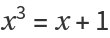:

 In:=Out=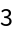Find the root degree for roots of polynomials of orders 2 to 8:

 In:=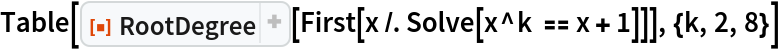Out=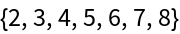Find the root degree of some trigonometric roots:

 In:=Out=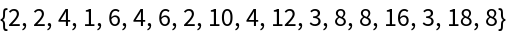In:=Out=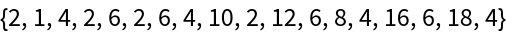### Scope (2)

Root degree of an AlgebraicNumber:

 In:=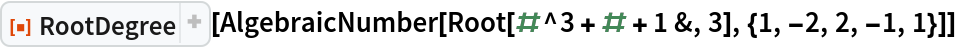Out=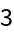Show the root degree of cumulative sums of roots of primes:

 In:=Out=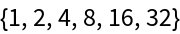### Possible Issues (3)

The default \$MaxRootDegree is 1000:

 In:=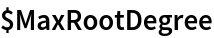Out=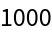Under default conditions, a degree-1000 root may be evaluated:

 In:=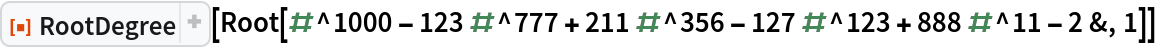Out=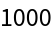A degree-1001 root will fail under default conditions:

 In:=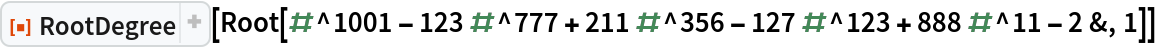Out=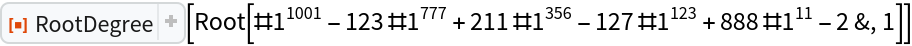RootDegree only works on exact algebraic numbers:

 In:=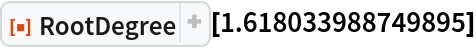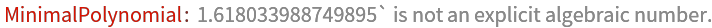Out=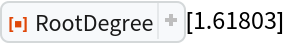Use RootApproximant to get a number that can be given to RootDegree:

 In:=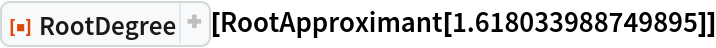Out=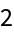Some algebraic numbers might not be recognized by RootDegree:

 In:=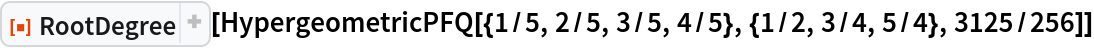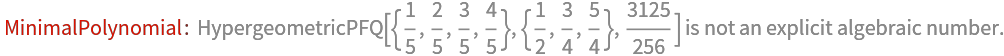Out=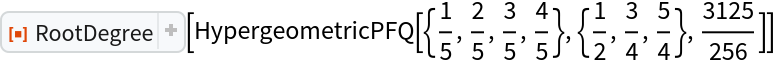In:=Out=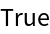## Version History

• 1.0.0 – 07 February 2022